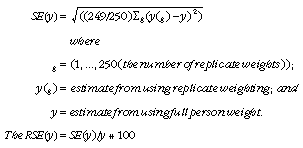4720.0 - National Aboriginal and Torres Strait Islander Social Survey: Users' Guide, 2008
ARCHIVED ISSUE Released at 11:30 AM (CANBERRA TIME) 26/02/2010
 Page tools: .mffeedback,#pjs { display :none; } Enable Javascript to Print PagesPrint All Glossary Abbreviations Questionnaire flow charts (Appendix) Replicate weights technique (Appendix) Data Cubes (I-Note) APPENDIX 2 REPLICATE WEIGHTS TECHNIQUE OVERVIEW A class of techniques called replication methods provide a general process of estimating variances for the types of complex sample designs and weighting procedures employed in ABS household surveys. A basic idea behind the replication approach is to select subsamples repeatedly from the whole sample. For each of these subsamples the statistic of interest is calculated. The variance of the full sample statistic is then estimated using the variability among the replicate statistics calculated from these subsamples. The subsamples are called replicate groups and the statistics calculated from these replicates are called replicate estimates. There are various ways of creating replicate subsamples from the full sample. The replicate weights produced for the 2008 National Aboriginal and Torres Strait Islander Social Survey (NATSISS) have been created under the delete-a-group jackknife method of replication which is described below. Some of the advantages in using replicate weights are that: the same process is applicable to most statistics, such as means, percentages, ratios, correlations, derived statistics and regression coefficients; and it is not necessary to have detailed survey design information, if the replicate weights are included with the survey data file. DERIVATION OF REPLICATE WEIGHTS The delete-a-group jackknife method of replicate weighting was used to derive weights, through the following process: 250 replicate groups were formed with each group formed to mirror the overall sample. Units from a Collection District (CD) all belong to the same replicate group and a unit can belong to only one replicate group; one replicate group was dropped from the file and then the remaining records were weighted in the same manner as for the full sample; the records in that group that was dropped received a weight of zero; this process was repeated for each replicate group (i.e. a total of 250 times); and each record had 250 replicate weights attached to it with one of these being the zero weight. APPLICATION OF REPLICATE WEIGHTS As noted above, replicate weights enable variances of estimates to be calculated relatively simply. They also enable unit records analyses such as chi-square and logistic regression to be conducted, which take into account the sample design. Replicate weights for any variable of interest can be calculated from the 250 replicate groups, giving 250 replicate estimates. The distribution of this set of replicate estimates, in conjunction with the full sample estimate (based on the general weight) is then used to approximate the variance of the full sample. The formula for calculating the Standard error (SE) and relative standard error (RSE) of an estimate using this method is shown below.This method can also be used when modelling relationships from unit record data, regardless of the modelling technique used. In modelling, the full sample would be used to estimate the parameter being studied, such as a regression co-efficient, the 250 replicate groups used to provide 250 replicate estimates of the survey parameter. The variance of the estimate of the parameter from the full sample is then approximated, as above, by the variability of the replicate estimates. USE OF REPLICATE WEIGHTS WITH STATISTICAL PACKAGES Not all statistical computer packages may allow direct calculation of SEs using the delete-a-group jackknife replicate weights. However, those packages that allow the direct use of Balanced Repeated Replication (BRR) methodology generally include the option of an adjustment factor. This factor can be incorporated to overcome the difference between the variance formulae. AVAILABILITY OF RSES CALCULATED USING REPLICATE WEIGHTS Actual RSEs were used in the summary publication released from this survey. The RSEs for estimates published in the National Aboriginal and Torres Strait Islander Social Survey, 2008 (cat. no. 4714.0) are available from the ABS website in spreadsheet format. The RSEs in the spreadsheets were calculated using the replicate weights methodology. Other RSEs for survey estimates are available on request. For more information see the Survey products chapter. Document Selection These documents will be presented in a new window.